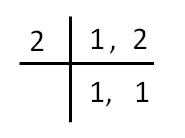Comparing ratios

Chapter 7 Class 7 Comparing Quantities
Concept wise

Let’s check which ratio is greater

2 : 1 or 1 : 2

To check,

we write ratio in fraction form

2/1 or 1/2

So, we have to find greater of  2/1 & 1/2

First, we make denominator same

Common denominator = LCM of 1 & 2

= 2

Thus,

2/1  = 2/1 × 2/2  = 4/2

1/2  = 1/2So,

4/2 > 1/2

i.e.  2/1 > 1/2

2 : 1 is greater ratio

#### Compare 4 : 2 & 3 : 2

Writing ratio in fraction form

4/2 & 3/2

Here denominator are same

Now, number with greater numerator is greater,

4/2 > 3/2

∴ 4 : 2 > 3 : 2

So, 4 : 2 is the greater ratio

#### Compare 7 : 3 & 5 : 2

Writing ratio in fraction form

7/3 & 5/2

First, we have to make denominator same,

Common denominator = L.C.M of 3 & 2

= 2 × 3

= 6

Thus,

7/3       = 7/3 × 2/2       = 14/6

5/2       = 5/2 × 3/3       = 15/6

So,

14/6 < 15/6

7 : 3 < 5 : 2

So , 5 : 2 is the greater ratio

Learn in your speed, with individual attention - Teachoo Maths 1-on-1 Class

### Transcript

Common denominator = LCM of 1 & 2 = 2 Thus, 2/1 2/1 × 2/2 = 4/2 1/2 So, 4/2 > 1/2 i.e. 2/1 > 1/2 ∴ 2 : 1 is greater ratio